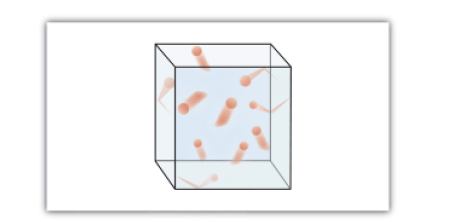# Kinetic Molecular Theory of Gases | Kinetic theory of gases

The kinetic molecular theory of gases is a model that helps us understand the physical properties of gases at the molecular level. This kinetic theory of gases describes this state of matter as composed of tiny particles in constant motion with a lot of distance between the particles.

The kinetic molecular theory of gases is based on the following concepts:

1. Gases consist of particles (molecules or atoms) that are in constant random motion.
2. Gas particles are constantly colliding with each other and the walls of their container. These collisions are elastic; that is, there is no net loss of energy from the collisions.
3. Gas particles are small and the total volume occupied by gas molecules is negligible relative to the total volume of their container.
4. There are no interactive forces (i.e., attraction or repulsion) between the particles of a gas.
5. The average kinetic energy of gas particles is proportional to the absolute temperature of the gas, and all gases at the same temperature have the same average kinetic energy.

Figure 1 “The Kinetic Molecular Theory of Gases” shows a representation of how we mentally picture the gas phase.Figure 1 “The Kinetic Molecular Theory of Gases.” The kinetic molecular theory of gases describes this state of matter as composed of tiny particles in constant motion with a lot of distance between the particles.

Because most of the volume occupied by a gas is empty space, a gas has a low density and can expand or contract under the appropriate influence.

The fact that gas particles are in constant motion means that two or more gases will always mix as the particles from the individual gases move and collide with each other.

The number of collisions the gas particles make with the walls of their container and the force with which they collide determine the magnitude of the gas pressure.

Read also: kinetic theory of matter

Kinetic Molecular Theory of Gases | Kinetic theory of gases
Scroll to top
error: physicsTeacher.in